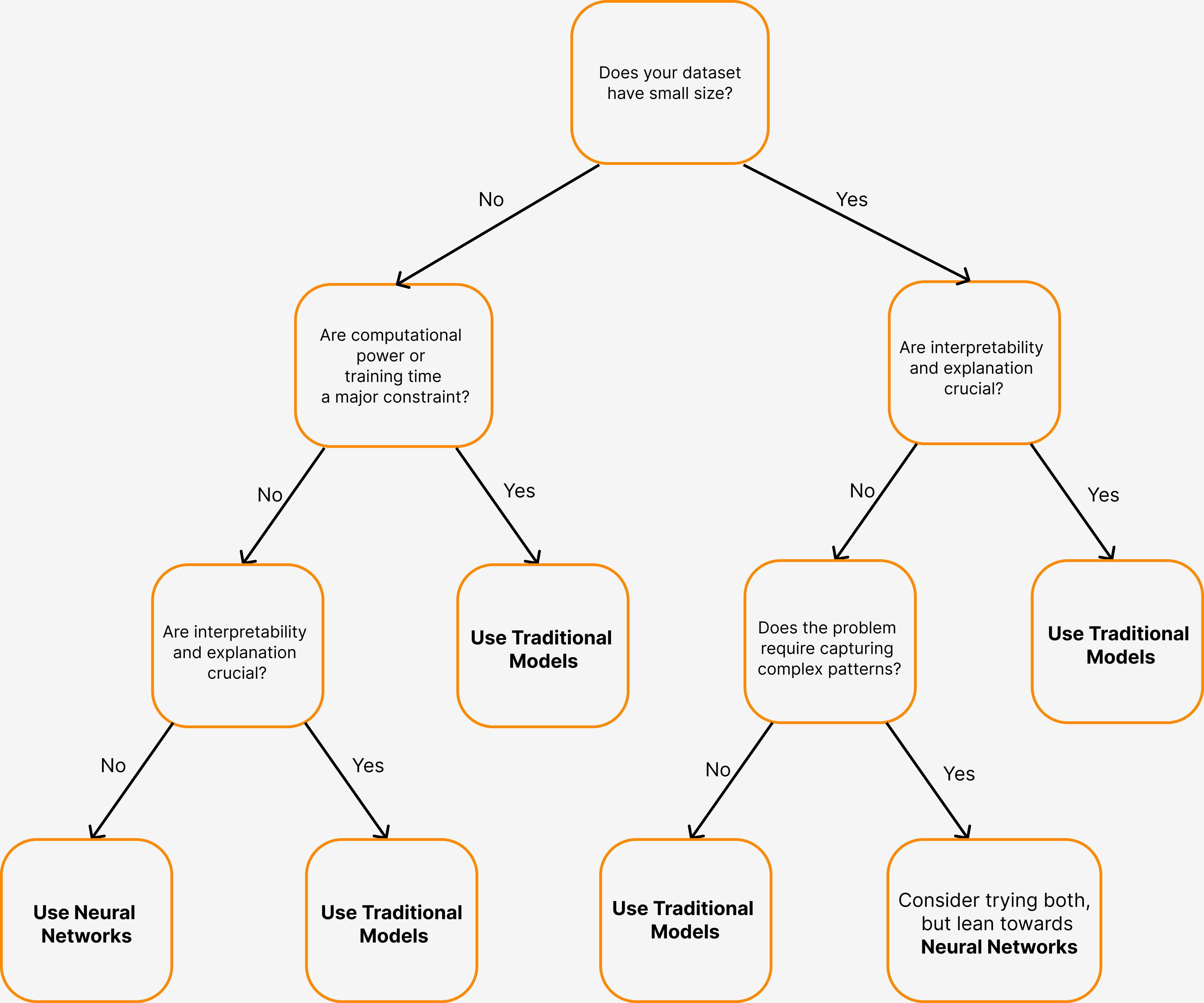Course Content

# Introduction to Neural Networks

Introduction to Neural Networks

## Concept of a Neural Network

Neuron is the basic unit of information processing.

Weights are special coefficients that determine how important each input is to the neuron.

The process of training a neural network is to adjust the weight of each neuron in such a way that the results they give are the most accurate.

The activation function converts the sum to the neuron's output value.

Examples of activation functions include:

1. Sigmoid Function
2. ReLU
3. Hyperbolic Tangent

Forward propagation is the process by which information passes through the Neural Network from the input layer to the output layer. When the information reaches the output layer, the network makes a prediction or inference based on the data it has processed.

Backpropagation is the process in which the error information is used to traverse the network back and adjust the weights of the neurons.

## Neural Network from Scratch

The bias allows the neuron to shift its output, adding flexibility to the modeling capability. Bias of the neuron is also a trainable parameter.

Multilayer Perceptron can have multiple layers:

1. An input layer: It receives the input data.
2. Hidden layers: These layers process the data and extract patterns.
3. Output layer: Produces the final prediction or classifications.

Each layer consists of multiple neurons, and the output from one layer becomes the input for the next layer.

We can split backpropagation algorithm into several steps:

1. Forward Propagation
2. Error Computing
4. Modifying Weights and Biases (Taking a Step in Gradient Descent)

Learning rate is an integral component of the gradient descent algorithm, the learning rate can be visualized as the pace of training. A higher learning rate accelerates the training process; however, an excessively high rate might cause the neural network to overlook valuable insights and patterns within the data.

There are many different ways to calculate the quality of a model:

1. Accuracy
2. Mean Squared Error (MSE)
3. Cross-entropy (Cross-entropy)
4. And many others...

Creating a model:

Training a model:

Predict output values:

## Conclusion

What to look for when choosinge between the Traditional Models and the Neural Networks:

1. Dataset Size
2. Complexity of a Problem
3. Interpretability
4. ResourcesThe most commonly used types of neural networks:

1. Feedforward Neural Networks (FNN) or Multi-layer Perceptrons (MLP)
2. Convolutional Neural Networks (CNN)
3. Recurrent Neural Networks (RNN)
4. Autoencoders (AE)
6. Modular Neural Networks (MNN)

Libraries for Deep Learning:

1. Tensorflow
2. PyTorchDownload Course Summary